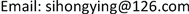﻿ 柯西积分公式的一点注记 A Note on Cauchy Integral Formula

Pure Mathematics
Vol. 09  No. 03 ( 2019 ), Article ID: 30088 , 5 pages
10.12677/PM.2019.93037

A Note on Cauchy Integral Formula

Hongying Si

College of Math Mathematics and Statistics, Shangqiu Normal University, Shangqiu HenanReceived: Apr. 14th, 2019; accepted: Apr. 25th, 2019; published: May 6th, 2019ABSTRACT

In this paper, according to integral calculation based on ${\int }_{c}\frac{1}{{\left(z-{z}_{0}\right)}^{n}}dz$ in Example 3.2, the integral value of Example 3.2 is calculated by the parametric equation method and the case 3.2 is generalized from the integral curve and the integrand function. First, the integral curve is generalized, and the circle with ${z}_{0}$ as the center and r as the radius is generalized to any closed curve containing ${z}_{0}$ ; after the promotion, this example has a wider scope of application. Secondly, the integrand function is promoted, $\frac{1}{z-{z}_{0}}$ is promoted to $\frac{f\left(z\right)}{z-{z}_{0}}$ and $\frac{f\left(z\right)}{{\left(z-{z}_{0}\right)}^{n+1}}$ respectively, the close relationship between the case 3.2 and the Cauchy integral formula and the high-order derivative formula of the analytic function is discussed further.

Keywords:Integral Curve, Cauchy Integral Formula, Higher Derivative FormulaCopyright © 2019 by author(s) and Hans Publishers Inc.1. 引言

2. 回顾例3.2

${\int }_{c}f\left(z\right)\text{d}z={\int }_{c}u\text{d}x-v\text{d}y+i{\int }_{c}v\text{d}x+u\text{d}y.$ (1)

$\begin{array}{c}{\int }_{c}f\left(z\right)\text{d}z={\int }_{c}\left(u+iv\right)\text{d}\left(x+iy\right)\\ ={\int }_{c}\left(u+iv\right)\left(\text{d}x+i\text{d}y\right)\\ ={\int }_{c}u\text{d}x+iu\text{d}y+iv\text{d}x-v\text{d}y\\ ={\int }_{c}u\text{d}x-v\text{d}y+i{\int }_{c}v\text{d}x+u\text{d}y\end{array}$

$\begin{array}{c}{\int }_{c}f\left(z\right)\text{d}z={\int }_{c}u\text{d}x-v\text{d}y+i{\int }_{c}v\text{d}x+u\text{d}y\\ ={\int }_{\alpha }^{\beta }\left[u\left(t\right){x}^{\prime }\left(t\right)-v\left(t\right){y}^{\prime }\left(t\right)\right]\text{d}t+i{\int }_{\alpha }^{\beta }\left[u\left(t\right){y}^{\prime }\left(t\right)+v\left(t\right){x}^{\prime }\left(t\right)\right]\text{d}t\end{array}$

${\int }_{c}f\left(z\right)\text{d}z={\int }_{\alpha }^{\beta }f\left[z\left(t\right)\right]{z}^{\prime }\left(t\right)\text{d}t,$ (2)

${\int }_{c}f\left(z\right)\text{d}z={\int }_{\alpha }^{\beta }\mathrm{Re}\left\{f\left[z\left(t\right)\right]{z}^{\prime }\left(t\right)\right\}\text{d}t+i{\int }_{\alpha }^{\beta }\mathrm{Im}\left\{f\left[z\left(t\right)\right]{z}^{\prime }\left(t\right)\right\}\text{d}t.$ (3)

$\begin{array}{c}{\int }_{c}\frac{1}{{\left(z-{z}_{0}\right)}^{n}}\text{d}z={\int }_{0}^{\text{2π}}\frac{ir{\text{e}}^{i\theta }}{{r}^{n}{\text{e}}^{in\theta }}\text{d}\theta =\frac{i}{{r}^{n-1}}{\int }_{0}^{\text{2π}}{\text{e}}^{-i\left(n-1\right)\theta }\text{d}\theta \\ =\frac{i}{{r}^{n-1}}{\int }_{0}^{\text{2π}}\mathrm{cos}\left(n-1\right)\theta \text{d}\theta +\frac{1}{{r}^{n-1}}{\int }_{0}^{\text{2π}}\mathrm{sin}\left(n-1\right)\theta \text{d}\theta \\ =\left\{\begin{array}{l}2\text{π}i,\text{\hspace{0.17em}}\text{\hspace{0.17em}}\text{\hspace{0.17em}}n=1;\\ 0,\text{\hspace{0.17em}}\text{\hspace{0.17em}}\text{\hspace{0.17em}}\text{\hspace{0.17em}}\text{\hspace{0.17em}}\text{\hspace{0.17em}}n\ne 1.\end{array}\end{array}$

3. 例3.2的推广形式

3.1. 将积分曲线推广

${\int }_{c}f\left(z\right)\text{d}z=\underset{k=1}{\overset{n}{\sum }}{\int }_{{c}_{k}}f\left(z\right)\text{d}z$

${\int }_{c}\frac{1}{{\left(z-{z}_{0}\right)}^{n}}\text{d}z={\int }_{{c}_{1}}\frac{1}{{\left(z-{z}_{0}\right)}^{n}}\text{d}z$

${\int }_{c}\frac{1}{{\left(z-{z}_{0}\right)}^{n}}\text{d}z={\int }_{{c}_{1}}\frac{1}{{\left(z-{z}_{0}\right)}^{n}}\text{d}z=\left\{\begin{array}{l}2\text{π}i\text{\hspace{0.17em}}\text{\hspace{0.17em}}\text{\hspace{0.17em}}n=1,\\ 0\text{\hspace{0.17em}}\text{\hspace{0.17em}}\text{\hspace{0.17em}}\text{\hspace{0.17em}}\text{\hspace{0.17em}}\text{\hspace{0.17em}}n\ne 1.\end{array}$

3.2. $n=1$ 时，将被积函数 $\frac{1}{z-{z}_{0}}$ 推广到 $\frac{f\left(z\right)}{z-{z}_{0}}$

$f\left({z}_{0}\right)=\frac{1}{2\text{π}i}{\oint }_{c}\frac{f\left(z\right)}{z-{z}_{0}}\text{d}z$

${\oint }_{c}\frac{f\left(z\right)}{z-{z}_{0}}\text{d}z=2\text{π}if\left(z0\right)$

3.3. $n\ne 1$ 时，将被积函数 $\frac{1}{{\left(z-{z}_{0}\right)}^{n}}$ 推广到 

${f}^{\left(n\right)}\left({z}_{0}\right)=\frac{n!}{2\text{π}i}{\oint }_{c}\frac{f\left(z\right)}{{\left(z-{z}_{0}\right)}^{n+1}}\text{d}z$ $\left(n=1,2,\cdots \right)$

${\oint }_{c}\frac{f\left(z\right)}{{\left(z-{z}_{0}\right)}^{n+1}}\text{d}z=\frac{2\text{π}i}{n!}{f}^{\left(n\right)}\left({z}_{0}\right).$

A Note on Cauchy Integral Formula[J]. 理论数学, 2019, 09(03): 282-286. https://doi.org/10.12677/PM.2019.93037

1. 1. 徐文莉. 复变函数积分计算与级数展开的教学探讨[J]. 考试周刊, 2015(95): 47-48.

2. 2. 王文鹏, 阙建华. 复变函数积分求解策略[J]. 重庆科技学院学报, 2007, 9(4): 145-147.

3. 3. 吴白旺. 利用复积分计算一种特殊类型的定积分[J]. 科技创新导报, 2010(2): 241-243.

4. 4. 朱敏慧, 崔艳. 浅析复变函数积分的计算[J]. 科技视界, 2012(31): 46-47.

5. 5. 郑唯唯, 朱敏慧, 李泽, 周立娜, 李海洋. 复变函数与积分变换[M]. 西安: 西北工业大学出版社, 2011.

6. 6. 陈静, 贠书杰. 复变函数积分的几种计算方法[J]. 河南机电高等专科学校学报, 2013, 21(2): 21-24.

7. 7. 杨华军, 王仕璠, 郝智明, 等. 复变函数论典型环路积分的理论分析[J]. 四川大学学报(自然科学版), 2001(S1): 69-74.

8. 8. 黄得隆. 复变函数积分计算中的几种方法[J]. 宝鸡文理学院学报(自然科学版), 1995(2): 71-74.

9. 9. 王艳琴. 计算复积分的几种方法[J]. 湖南工业职业技术学院学报, 2011, 11(5): 8-11.

10. 10. 唐宝庆, 杨润生, 欧阳文, 李立军. 对复变函数积分 的计算在教学上的探讨[J]. 数学理论与应用, 2010(1): 120-122.

11. 11. 张洁萍. 复变函数方法的两个应用研究[J]. 黑龙江科学, 2017, 8(16): 38-39.

12. 12. 钟玉泉. 复变函数论[M]. 北京: 高等教育出版社, 2012.

13. 13. 李红, 谢松法. 复变函数与积分变换[M]. 北京: 高等教育出版社, 2013.